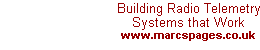Antenna Gain Explained

This one stumps even some of the most advanced RF engineers, that is, the "gain" of an antenna. Even the law states that the "Effective Radiated Power (ERP) will not exceed..." and this is based on the input into the antenna multiplied by the antenna gain. There is this concept that, the moment they exhibit gain, antennas magically create power within themselves. Sadly, this is not the case. If one examines an antenna it will be noted it is constructed of basic materials, the best being gold, silver, copper, then aluminium following on. These materials in themselves cannot create power.

Before we go into any explanations there are some terms that need definition so-as to assist in the explanation of antenna gain.

Coverage: The physical geological area where signal is still at a level which can be received, usually described as a radius distance from the antenna site.Radiation Pattern: A graphical representation of the intensity of the radiation vs. the angle from the perpendicular. The graph is usually circular, the intensity indicated by the distance from the centre based in the corresponding angle.

All radiation patterns on this page are with the antenna element(s) mounted vertically, and viewed from the side (i.e. right-angles to the antenna) as seen alongside.

decibel (dB): unit of measure of loss or gain, equal to 10*log(out/in) for power and 20*log(out/in) for voltage. Gain is a positive value, loss is a negative value.

Antenna Gain: The relative increase in radiation at the maximum point expressed as a value in dB above a standard, in this case the basic antenna, a ½-wavelength dipole (as in Two-Poles) by which all other antennas are measured. The reference is known as 0dBD (zero decibel referenced to dipole).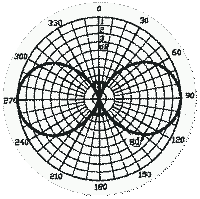To start with let us take a standard ½-wavelength dipole and "suspend" it in free space (i.e. ignore all possible surroundings e.g. the mounting pole etc. that could affect the antenna). The radiation pattern of this antenna is typically referred to as the "doughnut" as shown in the adjoining figure.
 As the materials cannot create power the only other alternative is to focus the wasted energy, for example that which is going skywards, towards a more useful direction being on the horizontal plane. The result is shown in the adjoining picture. Here the shape of the radiation was changed such that the outer most energy was focused to compliment the middle half, the result being a doubling of the radiated energy along the required plane or effectively a 3dB gain.This focusing can be even further intensified such that gains of 6dB (4 times) to 9dB (8 times) can be achieved. The resultant two patterns shown below.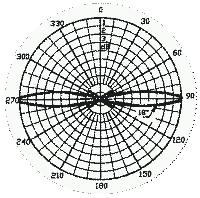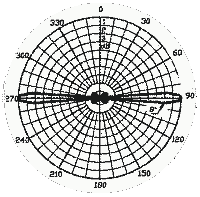As can be seen the method by which an antenna is made to have "gain" is merely to focus the radiation (i.e. flatten the doughnut shape) thus intensifying the radiation along the horizontal. Antennas with omni-directional radiation and gains of beyond 9dB are impractical owing to the fact that the focusing is directly related to the length (in wavelengths) of the antenna. There is, however, one further method of focusing, to now intensify the radiation in only one direction.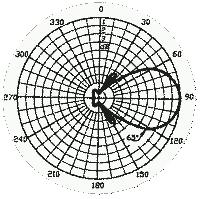If a reflector is placed next to a dipole all the energy that would have radiated in the direction of the reflector is now reflected back in the direction of the dipole. This makes all the energy appear in only one hemisphere and thus results in a doubling of radiated energy in this direction or 3dB gain.
 Further focusing can be achieved with the use of "directors" and again, by making the angle smaller and smaller i.e. packing all the radiation into one direction, higher gain is achieved. Here it is practical to achieve gains as high as 20dB. The effective angle, however, of such an antenna is small (typically ±10 degrees).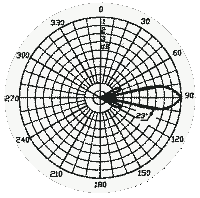As can be seen "gain" is also "loss". The higher the gain of an antenna the smaller the effective angle of use. This directly impacts the choice of the antenna for a specific function. Choosing the correct antenna is dealt with in a separate paper.

| | Ask a Question |

24.01.01